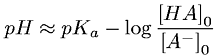Equations > Chemistry > Equilibrium > Buffer Design Equation

### Buffer Design EquationLatex Code:

MathML Code:

 $\mathrm{pH}\approx {\mathrm{pK}}_{a}-\mathrm{log}\frac{\left[\mathrm{HA}\right]}_{0}{\left[{A}^{-}\right]}_{0}$

MathType 5.0: